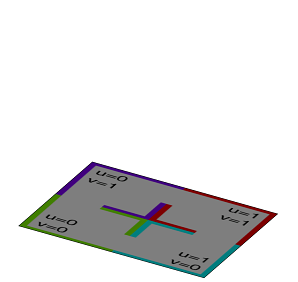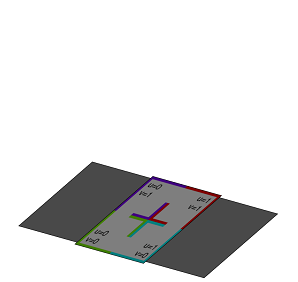1. widthfloat
2. lengthfloat

### Description

The primitiveQuad operation inserts a quad geometry into the scope of the current shape.

• If width and length aren’t given the bounding box coincides with the scope. Zero scope sizes are handled the same way as in the i operation.
• If dimensions are specified the quad is positioned in the xz-center of the scope lying on y=0.

If one scope size is zero while the 2 other sizes are not zero the quad will be inserted with respect to the plane defined by the valued scope sizes.

The quad has texture coordinates on the first texture layer (COLORMAP).

### Examples

 The inserted quad is fit into the current scope yz dimension. ``````Lot--> rotateScope(0, 0, 90) // scope.sx is 0 primitiveQuad() texture("builtin:uvtest.png")``````The inserted quad has a width of 10, a length of 20 and is positioned in the yz center. ``````Lot--> rotateScope(0, 0, 90) // scope.sx is 0 primitiveQuad(10,20) texture("builtin:uvtest.png")``````